# Angle + quadrilateral - math problems

#### Number of problems found: 23

• Diamond PQOR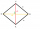In the diamond PQOR, the diagonal RQ is 4 cm long and the angle RPQ is 60°. What is the circumference of this diamond?
• Parallelogram ABCDWe have the parallelogram ABCD, where AB is 6.2 cm BC is 5.4 cm AC is 4.8 cm calculate the height on the AB side and the angle DAB
• ParallelogramFind the parallelogram's perimeter, where base a = 8 cm, height v = 3 cm, and angle alpha = 35° is the magnitude of the angle at vertex A.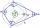What size have angles in quadrilateral (4-gon) if they are in a ratio of 8: 9: 10: 13?
• 4-gonIt is true that a 4-gon whose two sides are parallel and the other two has equal length, is a parallelogram?
• Interior angles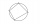In a quadrilateral ABCD, whose vertices lie on some circle, the angle at vertex A is 58 degrees and the angle at vertex B is 134 degrees. Calculate the sizes of the remaining interior angles.
• Mass point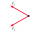Two equal forces of 30 Newtons act on a mass point. Find the magnitude of the resultant force if these forces form an angle of 42°.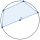A quadrilateral is inscribed in the circle so that its vertices divide the circle 1: 2: 3: 4. Find the sizes of its interior angles.What is the volume of a quadrilateral oblique prism with base edges of length a = 1m, b = 1.1m, c = 1.2m, d = 0.7m, if a side edge of length h = 3.9m has a deviation from the base of 20° 35' and the edges a, b form an angle of 50.5°.Show that the quadrilateral with vertices P1(0,1), P2(4,2) P3(3,6) P4(-5,4) has two right triangles.In a regular quadrilateral pyramid, the height is 6.5 cm and the angle between the base and the side wall is 42°. Calculate the surface area and volume of the body. Round calculations to 1 decimal place.The height of a regular quadrilateral prism is v = 10 cm, the deviation of the body diagonal from the base is 60°. Determine the length of the base edges, the surface, and the volume of the prism.How many square meters are needed to cover the shape of a regular quadrangular pyramid base edge 10 meters if the deviation lateral edges from the base plane are 68°? Calculate waste 10%.
• Digging a pitThe pit has the shape of a regular quadrilateral truncated pyramid. The edges of the bases are 14m and 10m long. The sidewalls form an angle of 135° with a smaller base. Determine how many m3 of soil were excavated when digging the pit?
• Tetrahedral pyramidCalculate the regular tetrahedral pyramid's volume and surface if the content area of the base is 20 cm2, and the deviation angle of the side edges from the plane of the base is 60 degrees.Calculate the volume (V) and the surface (S) of a regular quadrilateral prism whose height is 28.6 cm and the deviation of the body diagonal from the base plane is 50°.The regular quadrilateral pyramid has a base edge a = 1.56 dm and a height h = 2.05 dm. Calculate: a) the deviation angle of the sidewall plane from the base plane b) deviation angle of the side edge from the plane of the base
• 4side pyramidCalculate the volume and surface of the regular four-sided pyramid whose base edge is 4 cm long. The angle from the plane of the sidewall and base plane is 60 degrees.
• Angle of diagonalThe angle between the body diagonal of a regular quadrilateral and its base is 60°. The edge of the base has a length of 10cm. Calculate the body volume.In a regular quadrilateral pyramid, the side edge is e = 7 dm, and the diagonal of the base is 50 cm. Calculate the pyramid shell area.